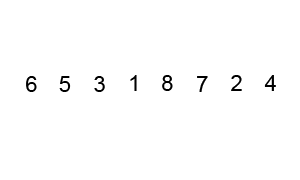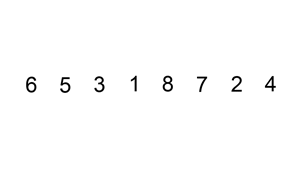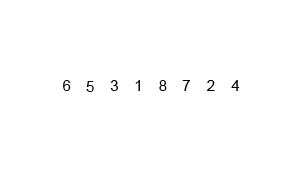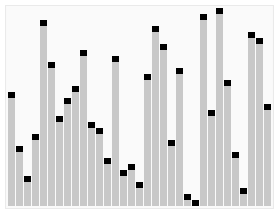# Sorting

#### sal/algo/sort.h

Comparison sorts (optimal runtime of `O(nlgn)`) Distribution sorts (optimal runtime of `O(n)`, requires "digit"-like substructure) partition reorder elements of a sequence either around a pivot or based on a predicate bub_sort sort a sequence by pairwise comparisons and swaps lin_sort sort a sequence by building onto a partially sorted sequence with linear search ins_sort sort a sequence by building onto a partially sorted sequence with binary search mer_sort sort a sequence by recursively merging sorted subsequences qck_sort sort a sequence by iteratively partitioning it in two heap_sort sort a sequence by modeling it as a heap and iteratively extracting top cnt_sort sort a sequence by counting the distribution of occurrances rdx_sort sort a sequence using counting sort on each digit tim_sort sort a sequence using insertion sort to create short, sorted subsequences that are then merged pat_sort sort a sequence by simulating solitaire

All sorting algorithms are called using iterators as shown below, unless shown otherwise. The parameters include the sequence [begin,end) where end is 1 element after the last.

### Partition ¶

Declaration

Parameters

 s set of sequences from which the intersection should be taken on

Return value

Iterator to the pivot or the first element that does not satisfy the predicate.

Example

Discussion

A very important function that enables the divide and conquer capability of other functions such as quicksort and quickselect. `n - 1` comparisons or calls of the unary predicate is done, resulting in `O(n)` time complexity. As the comparisons and swaps are performed in place (only requiring a constant amount of memory for swap), the space complexity is `O(1)`.

### Comparison sorts ¶

Comparison sorts are distinguished by their use of binary comparison functions such as `std::less` (two inputs of the same type and a boolean output) to sort sequences by doing pairwise comparisons. This requires a minimum amount of comparisons to retrieve the necessary information and has been proven to be `O(nlgn)`, with the implication of being the lower bound for all comparison sorting algorithms' average and worst case time.

The only assumption on the data type to be sorted is that it has be ordered - the "smaller" of two elements can be determined. This assumption is widley true and as a result comparison sorts are applicable to a wide range of data types.

### Bubble sort ¶

Declaration

Parameters

 begin iterator pointing to the first sequence element end iterator pointing to one past the last sequence element

Discussion

An inefficient `O(n^2)` algorithm that bubbles (compares and then shifts) smaller elements from the right to the left. Alternatives exist for bubbling larger elements from left to right with the same performance. That variation is described by the animation below:### Insertion sort ¶

Declaration

Discussion

A situational algorithm that depends on how far each element is away from their sorted position, having time complexity `O(nk)`, where all elements are at most k places from correct position. This property is exploited in hybrid sorts such as timsort, where insertion sort is applied on short, nearly sorted parts of the sequence.

The binary version is best suited for strings and other data types that have expensive comparisons relative to swaps. Otherwise, the linear shifting version performs better and is depicated in the animation below.### Merge sort ¶

Declaration

Discussion

A `O(nlgn)` algorithm that uses `O(n)` auxiliary memory. Pure merge sort is rarely used in practice due to its memory overhead, leading it to be many times slower than quicksort. However, it does illustrate the divide and conquer strategy very well, as shown in the animation below:### Quick sort ¶

Declaration

Discussion

A `O(nlgn)` algorithm that sorts in place significantly faster than any other pure comparison sort. It is both quick to sort and quick to implement (arguably the easiest `O(nlgn)` to implement). It relies on partitioning the sequence in two (`O(n)` operation) and then recursively sorting the two partitions.### Heap sort ¶

Declaration

Discussion

A heap is a datastructure that always has an easily accessible min or max value, with `O(lgn)` time to remake the heap after extracting the top element. Thus iteratively extracting the top element will result in a sorted extraction in `O(nlgn)` time. The image below illustrates the heap, which is usually internally represented as a linear array, but can be thought of as a complete binary tree:### Distribution sorts ¶

Distribution sorts have the strong assumption that the data type has a finite and known range. For exmaple, 16 bit shorts are constrained between -32768 to 32767, which has the finite range of 65536. Many data types do not have a limited range, such as character strings, and are not well suited to be sorted using distribution sorts.

However, with that strong assumption also comes a decrease in minimum bound, which is reduced to the optimal `O(n)`. It is not possible to be sublinear since each element has to be considered at least once.

### Counting sort ¶

Declaration

Parameters

 range size of the bin to use for holding all potential values op extraction operator that optionally looks at only a part of the data

Example

Discussion

Counting sort uses a direct address table (where the value is the key to a hashtable) to keep count of the occurances of each unique value. One pass through the original data fully constructs the table in `O(n)` time, assuming it takes `O(1)` time to address the table.

The sequence is then recreated in some kind of order implicit to the way the table is structured. The most basic form applied to non-positive integers would create a table where the index directly translates into the value, and iterating over the table from begin to end would recreate the original sequence sorted via `std::less` while iterating from end to begin would result in sorting via `std::greater`.

Declaration

Parameters

 bits the highest bit to consider if the data spans less than [min,max] range the size of the direct address table used for counting sort

Example

Discussion

Radix sort is an extension of counting sort that makes it much more practical for larger data types. Counting sort needs a direct address table to fit the entire range of the data, so for a data type with 32 bits, it'd need an array of 2^32 bits, which would be too large for most computers. Radix sort, on the other hand, can operate on arbitrary "digits" (in practice certain bits) of the data at a time, with the usual digit size being 8 bits = 1 byte. Counting sort can then be seen as a special case of radix sort with the digit being the size of the entire data type.

It runs in `O(nk)` time, where k is the number of digits in the data set corresponding to how many passes of counting sort will be done.

Radix sort comes in two variants differentiated by whether it starts on the most or least significant digit. Contrary to intuition, sorting from the least significant to most significant digit works, and has the advantage of being stable - "equal" elements maintain their relative position at each pass of the sort. Stability is generally preferred, but is especially important when the property being sorted is only part of the item being sorted (ex. people can be sorted on names, but they also have hobbies and favourite colours). Correspondingly, SAL implements on the least significant digit variant.

### Hybrid sorts ¶

Hybrid sorts are usually what is used as standard libraries' general sorts, such as C++'s `std::sort`, which is a variant called Introsort switching from quicksort to heap sort based on the recursion depth. They maintain the generality of comparison sorts but also provide a speedup relative to pure comparison sorts. A disadvantage is that they are harder to implement optimally, as there is usually upkeep associated with bookkeeping when to switch strategies.

### Tim sort ¶

Declaration

Parameters

 bits the highest bit to consider if the data spans less than [min,max] range the size of the direct address table used for counting sort

Example

Discussion

Radix sort is an extension of counting sort that makes it much more practical for larger data types. Counting sort needs a direct address table to fit the entire range of the data, so for a data type with 32 bits, it'd need an array of 2^32 bits, which would be too large for most computers. Radix sort, on the other hand, can operate on arbitrary "digits" (in practice certain bits) of the data at a time, with the usual digit size being 8 bits = 1 byte. Counting sort can then be seen as a special case of radix sort with the digit being the size of the entire data type.

It runs in `O(nk)` time, where k is the number of digits in the data set corresponding to how many passes of counting sort will be done.

Radix sort comes in two variants differentiated by whether it starts on the most or least significant digit. Contrary to intuition, sorting from the least significant to most significant digit works, and has the advantage of being stable - "equal" elements maintain their relative position at each pass of the sort. Stability is generally preferred, but is especially important when the property being sorted is only part of the item being sorted (ex. people can be sorted on names, but they also have hobbies and favourite colours). Correspondingly, SAL implements on the least significant digit variant.